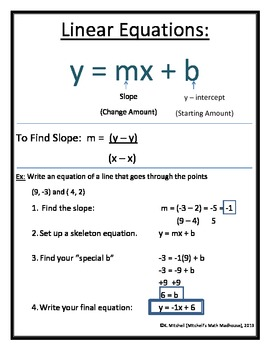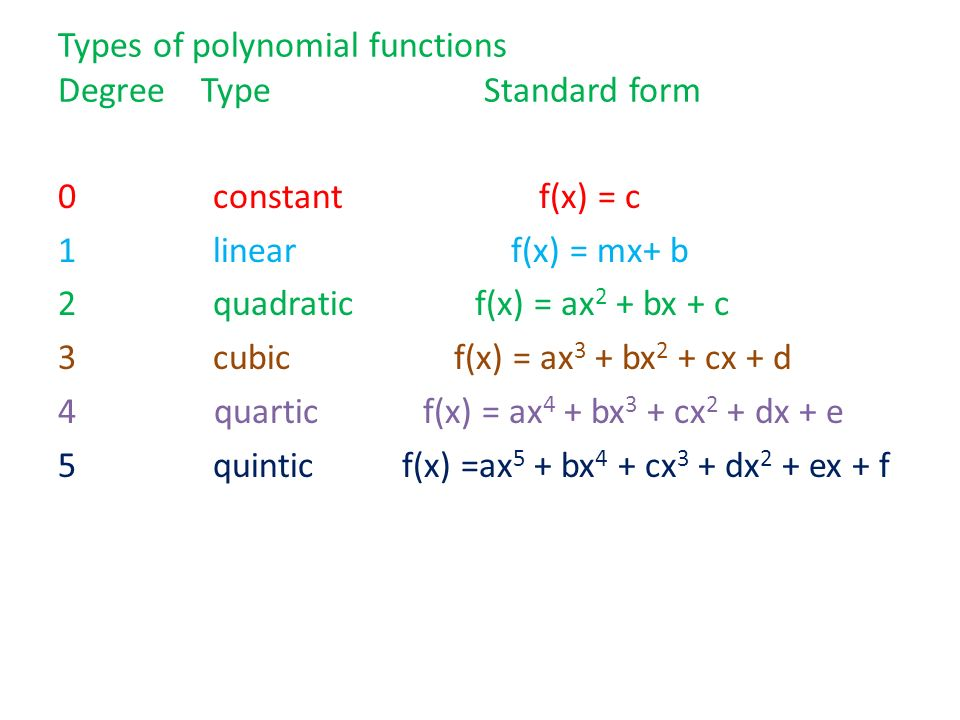# Algebral 1

In its most general form, algebra is the study of mathematical symbols and the rules for manipulating these symbols; it is a unifying thread of almost all of mathematics. As such, it includes everything from elementary equation solving to the study of abstractions such as groups, rings, and fields. AlgebraThe image has show a formula and what each means of linear equation. Next there will be more equation, that will show on the below:Practice for Algebal 1 and the answer to the practice is on the same link.